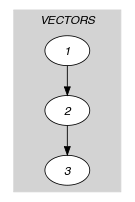## 5.223. lex_chain_greater

Origin
Constraint

$\mathrm{𝚕𝚎𝚡}_\mathrm{𝚌𝚑𝚊𝚒𝚗}_\mathrm{𝚐𝚛𝚎𝚊𝚝𝚎𝚛}\left(\mathrm{𝚅𝙴𝙲𝚃𝙾𝚁𝚂}\right)$

Usual name

$\mathrm{𝚕𝚎𝚡}_\mathrm{𝚌𝚑𝚊𝚒𝚗}$

Type
 $\mathrm{𝚅𝙴𝙲𝚃𝙾𝚁}$ $\mathrm{𝚌𝚘𝚕𝚕𝚎𝚌𝚝𝚒𝚘𝚗}\left(\mathrm{𝚟𝚊𝚛}-\mathrm{𝚍𝚟𝚊𝚛}\right)$
Argument
 $\mathrm{𝚅𝙴𝙲𝚃𝙾𝚁𝚂}$ $\mathrm{𝚌𝚘𝚕𝚕𝚎𝚌𝚝𝚒𝚘𝚗}\left(\mathrm{𝚟𝚎𝚌}-\mathrm{𝚅𝙴𝙲𝚃𝙾𝚁}\right)$
Restrictions
 $|\mathrm{𝚅𝙴𝙲𝚃𝙾𝚁}|\ge 1$ $\mathrm{𝚛𝚎𝚚𝚞𝚒𝚛𝚎𝚍}$$\left(\mathrm{𝚅𝙴𝙲𝚃𝙾𝚁},\mathrm{𝚟𝚊𝚛}\right)$ $\mathrm{𝚛𝚎𝚚𝚞𝚒𝚛𝚎𝚍}$$\left(\mathrm{𝚅𝙴𝙲𝚃𝙾𝚁𝚂},\mathrm{𝚟𝚎𝚌}\right)$ $\mathrm{𝚜𝚊𝚖𝚎}_\mathrm{𝚜𝚒𝚣𝚎}$$\left(\mathrm{𝚅𝙴𝙲𝚃𝙾𝚁𝚂},\mathrm{𝚟𝚎𝚌}\right)$
Purpose

For each pair of consecutive vectors ${\mathrm{𝚅𝙴𝙲𝚃𝙾𝚁}}_{i}$ and ${\mathrm{𝚅𝙴𝙲𝚃𝙾𝚁}}_{i+1}$ of the $\mathrm{𝚅𝙴𝙲𝚃𝙾𝚁𝚂}$ collection we have that ${\mathrm{𝚅𝙴𝙲𝚃𝙾𝚁}}_{i}$ is lexicographically strictly greater than ${\mathrm{𝚅𝙴𝙲𝚃𝙾𝚁}}_{i+1}$. Given two vectors, $\stackrel{\to }{X}$ and $\stackrel{\to }{Y}$ of $n$ components, $〈{X}_{0},\cdots ,{X}_{n-1}〉$ and $〈{Y}_{0},\cdots ,{Y}_{n-1}〉$, $\stackrel{\to }{X}$ is lexicographically strictly greater than $\stackrel{\to }{Y}$ if and only if ${X}_{0}>{Y}_{0}$ or ${X}_{0}={Y}_{0}$ and $〈{X}_{1},\cdots ,{X}_{n-1}〉$ is lexicographically strictly greater than $〈{Y}_{1},\cdots ,{Y}_{n-1}〉$.

Example
$\left(〈\mathrm{𝚟𝚎𝚌}-〈5,2,6,3〉,\mathrm{𝚟𝚎𝚌}-〈5,2,6,2〉,\mathrm{𝚟𝚎𝚌}-〈5,2,3,9〉〉\right)$

The $\mathrm{𝚕𝚎𝚡}_\mathrm{𝚌𝚑𝚊𝚒𝚗}_\mathrm{𝚐𝚛𝚎𝚊𝚝𝚎𝚛}$ constraint holds since:

• The first vector $〈5,2,6,3〉$ of the $\mathrm{𝚅𝙴𝙲𝚃𝙾𝚁𝚂}$ collection is lexicographically strictly greater than the second vector $〈5,2,6,2〉$ of the $\mathrm{𝚅𝙴𝙲𝚃𝙾𝚁𝚂}$ collection.

• The second vector $〈5,2,6,2〉$ of the $\mathrm{𝚅𝙴𝙲𝚃𝙾𝚁𝚂}$ collection is lexicographically strictly greater than the third vector $〈5,2,3,9〉$ of the $\mathrm{𝚅𝙴𝙲𝚃𝙾𝚁𝚂}$ collection.

Typical
 $|\mathrm{𝚅𝙴𝙲𝚃𝙾𝚁}|>1$ $|\mathrm{𝚅𝙴𝙲𝚃𝙾𝚁𝚂}|>1$
Arg. properties
• Contractible wrt. $\mathrm{𝚅𝙴𝙲𝚃𝙾𝚁𝚂}$.

• Suffix-extensible wrt. $\mathrm{𝚅𝙴𝙲𝚃𝙾𝚁𝚂}.\mathrm{𝚟𝚎𝚌}$ (add items at same position).

Usage

This constraint was motivated for breaking symmetry: more precisely when one wants to lexicographically order the consecutive columns of a matrix of decision variables. A further motivation is that using a set of lexicographic ordering constraints between two vectors does usually not allows to come up with a complete pruning.

Algorithm

A filtering algorithm achieving arc-consistency for a chain of lexicographical ordering constraints is presented in [BeldiceanuCarlsson02c].

Keywords
Arc input(s)

$\mathrm{𝚅𝙴𝙲𝚃𝙾𝚁𝚂}$

Arc generator
$\mathrm{𝑃𝐴𝑇𝐻}$$↦\mathrm{𝚌𝚘𝚕𝚕𝚎𝚌𝚝𝚒𝚘𝚗}\left(\mathrm{𝚟𝚎𝚌𝚝𝚘𝚛𝚜}\mathtt{1},\mathrm{𝚟𝚎𝚌𝚝𝚘𝚛𝚜}\mathtt{2}\right)$

Arc arity
Arc constraint(s)
$\mathrm{𝚕𝚎𝚡}_\mathrm{𝚐𝚛𝚎𝚊𝚝𝚎𝚛}$$\left(\mathrm{𝚟𝚎𝚌𝚝𝚘𝚛𝚜}\mathtt{1}.\mathrm{𝚟𝚎𝚌},\mathrm{𝚟𝚎𝚌𝚝𝚘𝚛𝚜}\mathtt{2}.\mathrm{𝚟𝚎𝚌}\right)$
Graph property(ies)
$\mathrm{𝐍𝐀𝐑𝐂}$$=|\mathrm{𝚅𝙴𝙲𝚃𝙾𝚁𝚂}|-1$

Graph model

Parts (A) and (B) of Figure 5.223.1 respectively show the initial and final graph associated with the Example slot. Since we use the $\mathrm{𝐍𝐀𝐑𝐂}$ graph property, the arcs of the final graph are stressed in bold. The $\mathrm{𝚕𝚎𝚡}_\mathrm{𝚌𝚑𝚊𝚒𝚗}_\mathrm{𝚐𝚛𝚎𝚊𝚝𝚎𝚛}$ constraint holds since all the arc constraints of the initial graph are satisfied.

##### Figure 5.223.1. Initial and final graph of the $\mathrm{𝚕𝚎𝚡}_\mathrm{𝚌𝚑𝚊𝚒𝚗}_\mathrm{𝚐𝚛𝚎𝚊𝚝𝚎𝚛}$ constraint(a) (b)
Signature

Since we use the $\mathrm{𝑃𝐴𝑇𝐻}$ arc generator on the $\mathrm{𝚅𝙴𝙲𝚃𝙾𝚁𝚂}$ collection the number of arcs of the initial graph is equal to $|\mathrm{𝚅𝙴𝙲𝚃𝙾𝚁𝚂}|-1$. For this reason we can rewrite $\mathrm{𝐍𝐀𝐑𝐂}=|\mathrm{𝚅𝙴𝙲𝚃𝙾𝚁𝚂}|-1$ to $\mathrm{𝐍𝐀𝐑𝐂}\ge |\mathrm{𝚅𝙴𝙲𝚃𝙾𝚁𝚂}|-1$ and simplify $\underline{\overline{\mathrm{𝐍𝐀𝐑𝐂}}}$ to $\overline{\mathrm{𝐍𝐀𝐑𝐂}}$.